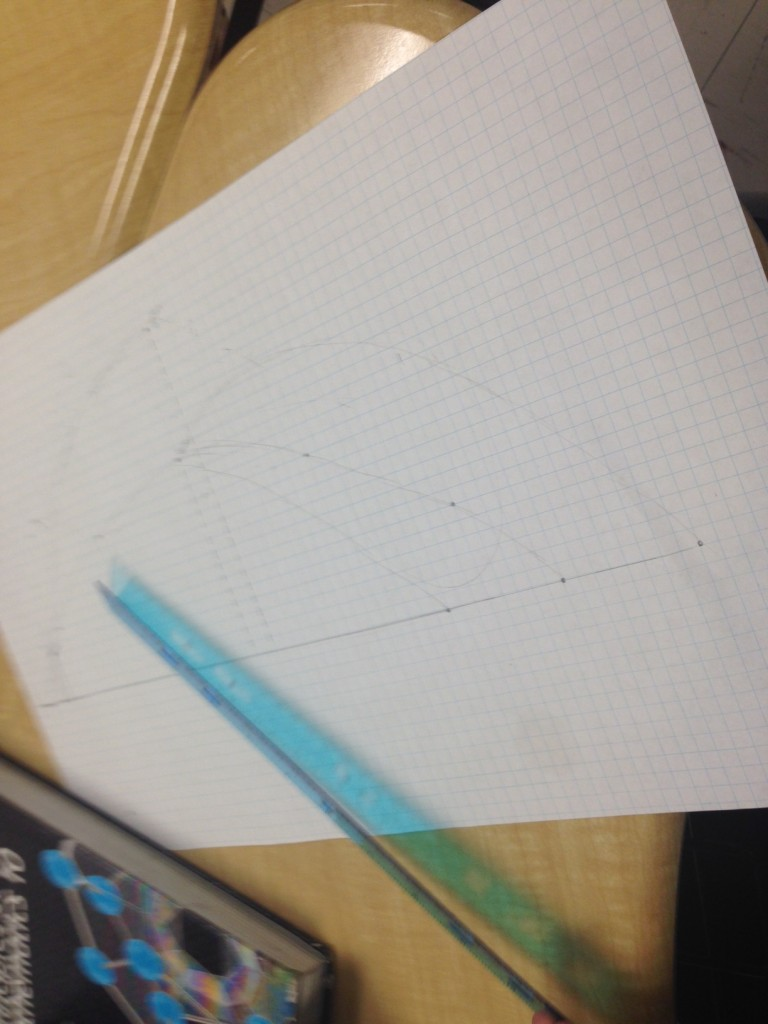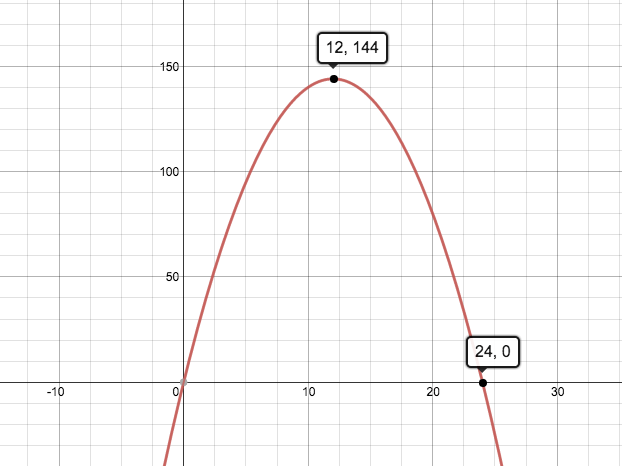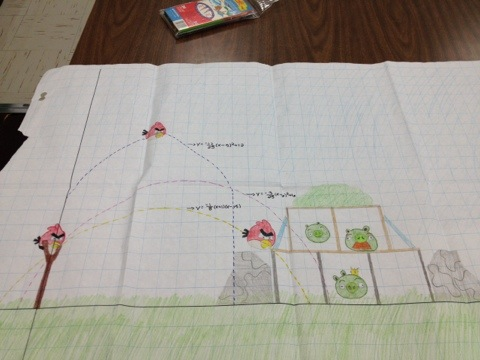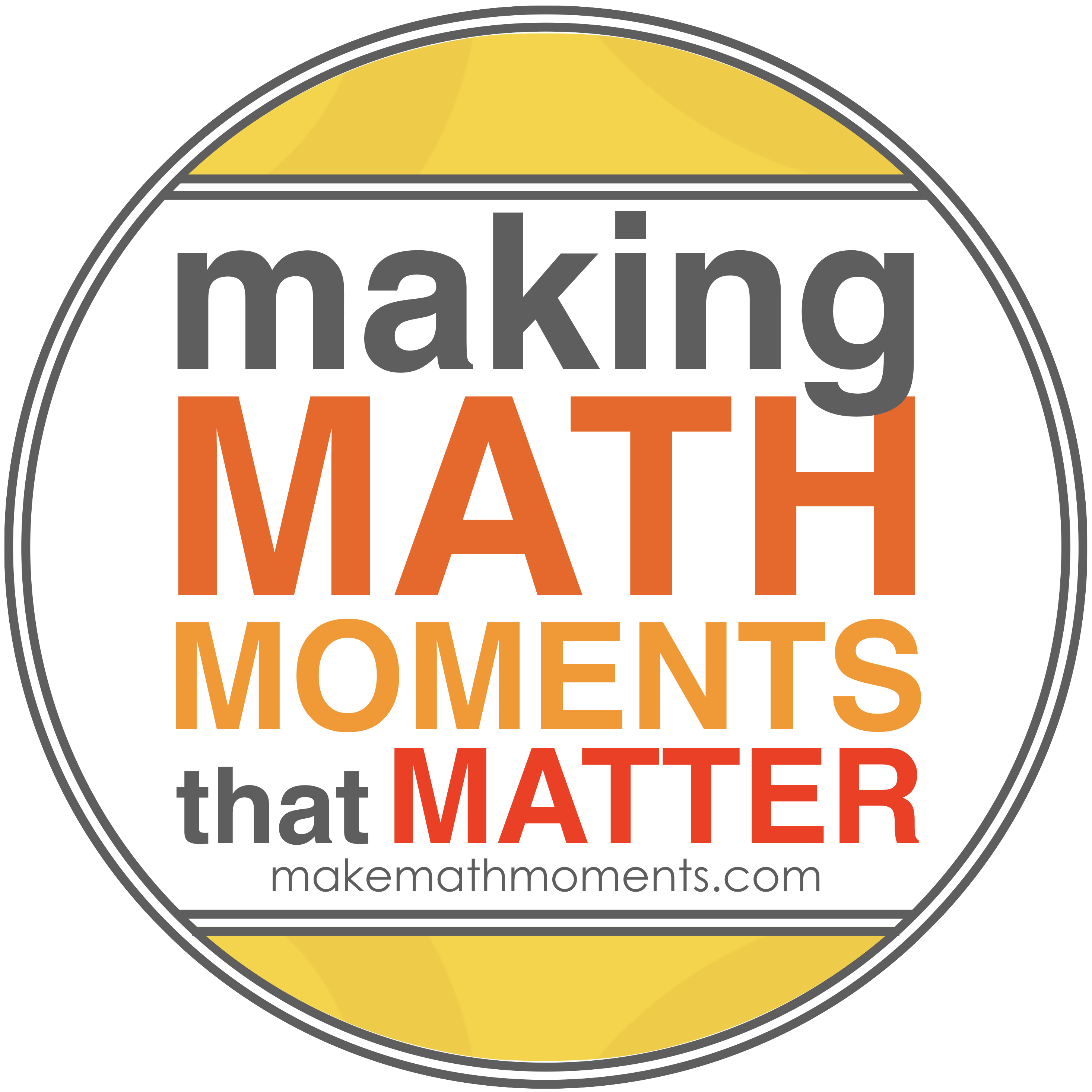# Angry Birds – Parabolas

[UPDATED – Sept. 25 2015]

We have just spent a week working with quadratics in MPM2D and today I had students create their own angry birds level.The only real requirement was for them to create at least two flight paths and model them with quadratic equations.

I was hoping to see how they relate the equation to the graph. This worked out better than expected. Most students did not initially make their curves symmetric…..and that gave us a chance to discuss symmetry and these questions

• Where would the zeros be?
• Where is your vertex? Where are the zeros in relation to your vertex?
• What could the equation be then?

Most students started creating their flight paths with placing the zeros on the graph….then picking a vertex. The best part of the day was discussing how students knew where their vertex was.Most knew that the axis of symmetry had to be half way between the zeros and also knew the vertex was on the axis. The issues, and then real thinking came when they went to create the equation to match. For example if Alexis wanted her bird to take off from x = 0 and land when x = 24 …she also wanted her highest height to be 7.5 units high. She wrote the equation y = – (x)(x – 24) and assumed she was correct. This assumption gave us lots to discuss!

I asked her to show me how she knew the vertex is (12,7.5) and she had a hard time. “I knew the x value was 12 but I just picked that height”. So I asked her to use the equation and find which height does go with an x value of 12. She did and then we graphed….Something clicked in her….”Aaah! The a-value must not just be -1″ and then that led us into discussing what the a-value should be and how we can get it more accurately! We did this…I loved that we discovered and struggled to find the correct a-value instead of me just giving an example and them copying.

I floated from group to group having similar conversations. Some students created their equations first and then found the vertex from the equation and plotted that.

Extensions

Some students started to ask if they could use different birds!!

• The blue bird once tapped would split off and create three more birds with three new flight paths.
• The yellow bird once tapped we assumed would make a straight line with a linear equation.

Pictures are from 2010….new pictures coming!!!## 5 thoughts on “Angry Birds – Parabolas”

1.Helen Crawford

This is awesome. I wonder, however, if it could be altered a bit so that students in Algebra 1 could do something similar. Our Alg 1 students are only familiar with the parts of the parabola and the zeros… they don’t get vertext form until Alg 2.. Ideas are very welcome!!!!

1.Mr. Orr

This definitely could be altered to only use the factored form equation. Students could design the level with flight paths based on the intercepts and a third point. You could discuss the idea of symmetry about the axis, halfway between the zeros.

2.Hi John, I love this idea. How did you support the students at the lower spectrum of your group?

3.Saefudin Zuchri

What an awesome idea. Can i use this for linear equation?## CONTACT ME